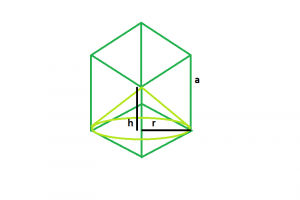# Largest cone that can be inscribed within a cube

Given here is a cube of side length a. We have to find the height and the radius of the biggest right circular cone that can be inscribed within it.

Examples:

```Input : a = 6
Output : r = 4.24264, h = 6

Input : a = 10
Output : r = 7.07107, h = 10
```## Recommended: Please try your approach on {IDE} first, before moving on to the solution.

Approach:
Let height of the cone = h.
and, radius of the cone = r.

From the diagram, we can clearly understand that,

• r = a/√2
• h = a

Below is the implementation of the above approach:

## C++

 `// C++ Program to find the biggest cone ` `// inscribed within a cube ` ` `  `#include ` `using` `namespace` `std; ` ` `  `// Function to find the radius of the cone ` `float` `coneRadius(``float` `a) ` `{ ` ` `  `    ``// side cannot be negative ` `    ``if` `(a < 0) ` `        ``return` `-1; ` ` `  `    ``// radius of the cone ` `    ``float` `r = a / ``sqrt``(2); ` `    ``return` `r; ` `} ` ` `  `// Function to find the height of the cone ` `float` `coneHeight(``float` `a) ` `{ ` `    ``// side cannot be negative ` `    ``if` `(a < 0) ` `        ``return` `-1; ` ` `  `    ``// height of the cone ` `    ``float` `h = a; ` `    ``return` `h; ` `} ` ` `  `// Driver code ` `int` `main() ` `{ ` `    ``float` `a = 6; ` ` `  `    ``cout << "r = " << coneRadius(a) << ", " ` `         ``<< "h = " << coneHeight(a) << endl; ` ` `  `    ``return` `0; ` `} `

## Java

 `// Java Program to find the biggest  ` `// cone inscribed within a cube ` `import` `java.util.*; ` `import` `java.lang.*; ` ` `  `class` `GFG ` `{ ` `// Function to find the radius  ` `// of the cone ` `static` `float` `coneRadius(``float` `a) ` `{ ` ` `  `    ``// side cannot be negative ` `    ``if` `(a < ``0``) ` `        ``return` `-``1``; ` ` `  `    ``// radius of the cone ` `    ``float` `r = (``float``)(a / Math.sqrt(``2``)); ` `    ``return` `r; ` `} ` ` `  `// Function to find the height  ` `// of the cone ` `static` `float` `coneHeight(``float` `a) ` `{ ` `    ``// side cannot be negative ` `    ``if` `(a < ``0``) ` `        ``return` `-``1``; ` ` `  `    ``// height of the cone ` `    ``float` `h = a; ` `    ``return` `h; ` `} ` ` `  `// Driver code ` `public` `static` `void` `main(String args[]) ` `{ ` `    ``float` `a = ``6``; ` ` `  `    ``System.out.println(``"r = "` `+ coneRadius(a) +  ` `                    ``", "` `+ ``"h = "` `+ coneHeight(a)); ` `} ` `} ` ` `  `// This code is contributed  ` `// by Akanksha Rai `

## Python 3

 `# Python 3 Program to find the biggest  ` `# cone inscribed within a cube ` `import` `math ` ` `  `# Function to find the radius  ` `# of the cone ` `def` `coneRadius(a): ` ` `  `    ``# side cannot be negative ` `    ``if` `(a < ``0``): ` `        ``return` `-``1` ` `  `    ``# radius of the cone ` `    ``r ``=` `a ``/` `math.sqrt(``2``) ` `    ``return` `r ` ` `  `# Function to find the height of the cone ` `def` `coneHeight(a): ` ` `  `    ``# side cannot be negative ` `    ``if` `(a < ``0``): ` `        ``return` `-``1` ` `  `    ``# height of the cone ` `    ``h ``=` `a ` `    ``return` `h ` ` `  `# Driver code ` `if` `__name__ ``=``=` `"__main__"``: ` `     `  `    ``a ``=` `6` ` `  `    ``print``(``"r = "``, coneRadius(a) ,  ` `          ``"h = "``, coneHeight(a)) ` ` `  `# This code is contributed by ChitraNayal `

## C#

 `// C# Program to find the biggest  ` `// cone inscribed within a cube ` `using` `System; ` ` `  `class` `GFG ` `{ ` `// Function to find the radius  ` `// of the cone ` `static` `float` `coneRadius(``float` `a) ` `{ ` ` `  `    ``// side cannot be negative ` `    ``if` `(a < 0) ` `        ``return` `-1; ` ` `  `    ``// radius of the cone ` `    ``float` `r = (``float``)(a / Math.Sqrt(2)); ` `    ``return` `r; ` `} ` ` `  `// Function to find the height  ` `// of the cone ` `static` `float` `coneHeight(``float` `a) ` `{ ` `    ``// side cannot be negative ` `    ``if` `(a < 0) ` `        ``return` `-1; ` ` `  `    ``// height of the cone ` `    ``float` `h = a; ` `    ``return` `h; ` `} ` ` `  `// Driver code ` `public` `static` `void` `Main() ` `{ ` `    ``float` `a = 6; ` ` `  `    ``Console.WriteLine(``"r = "` `+ coneRadius(a) +  ` `                       ``", "` `+ ``"h = "` `+ coneHeight(a)); ` `} ` `} ` ` `  `// This code is contributed  ` `// by Akanksha Rai `

## PHP

 ` `

Output:

```r = 4.24264, h = 6
```

Attention reader! Don’t stop learning now. Get hold of all the important DSA concepts with the DSA Self Paced Course at a student-friendly price and become industry ready.

My Personal Notes arrow_drop_upCheck out this Author's contributed articles.

If you like GeeksforGeeks and would like to contribute, you can also write an article using contribute.geeksforgeeks.org or mail your article to contribute@geeksforgeeks.org. See your article appearing on the GeeksforGeeks main page and help other Geeks.

Please Improve this article if you find anything incorrect by clicking on the "Improve Article" button below.

Article Tags :
Practice Tags :

Be the First to upvote.

Please write to us at contribute@geeksforgeeks.org to report any issue with the above content.